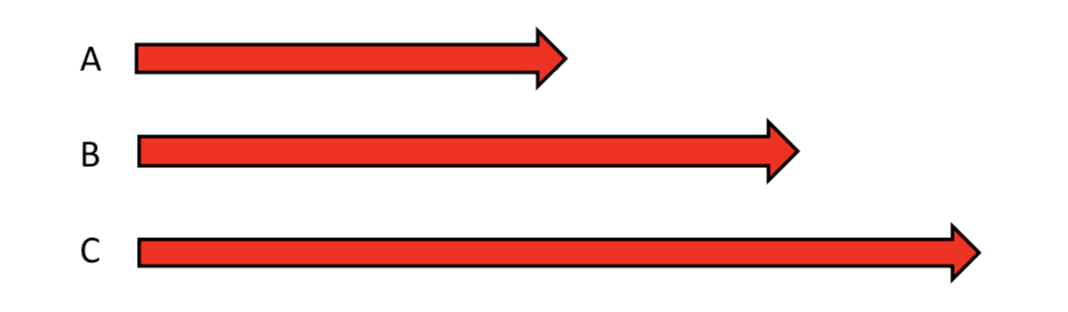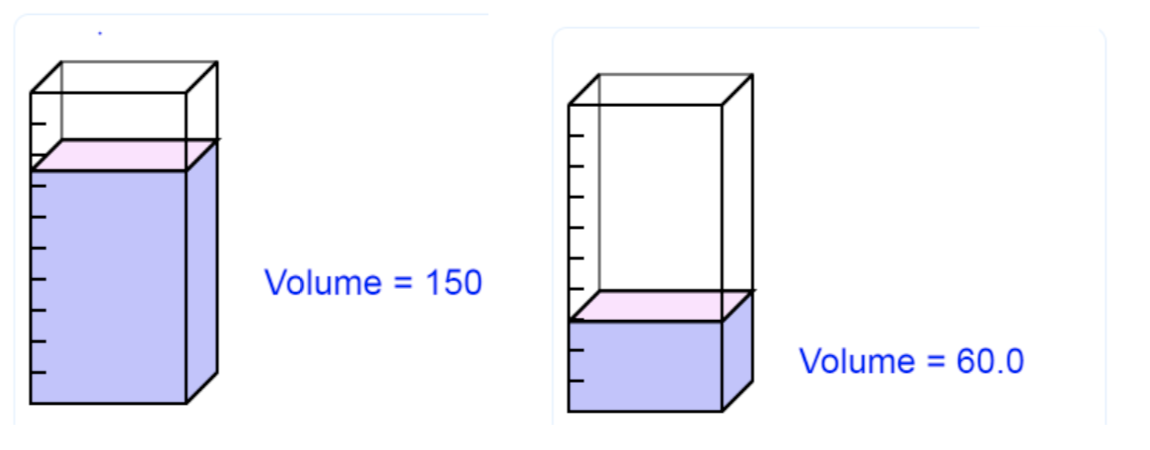# Numeracy for all (VSO)

Marilyn Leask, Emma Goto, Mary Watkins, Richard Procter | View as single page | Feedback/Impact

## Measurement

Written by Jane Jones (University of Winchester) and Clare Tope (Open University)

## 1.0 Overview

One of the most common ways in which we use numbers in everyday life is in measurement. Measure provides a concise way of describing an attribute using a number and a unit of measure and in doing so allows us to communicate lengths, weights, temperature and so on.

Children may have experience of comparing and measuring before they come to school in both standard and non-standard units. For example deciding which cake is bigger or another child is taller or shorter than themselves. It is important that skills and concepts are developed in tandem. For example there is no point in children being able to do complicated conversions between metres and centimetres if they are unable to estimate how long a metre or a centimetre is. By the end of the primary school children should know about the practical reasons why standard measures are used and be able to make judgments about the degree of accuracy that is needed in different situations. Learners should develop their measuring skills and understanding through a range of purposeful and practical activities in a range of subjects (Cross and Brothwick, 2016).

Analysis of common errors in measure indicate that mathematical thinking about measures remain tied to each measure itself and there is a lack in thinking about what is involved in the act of measurement (Ryan and Williams, 2007). To move beyond this stage Hansen (2017) recommends that teachers should focus on what it means to measure rather than what to measure. One way to do this is to focus on the principles which underpin all measures which are explored in detail.

https://www.cambridgemaths.org/espresso/view/effective-teaching-of-early-measurement/

## 2.0 Key Ideas

### 2.1 Principles of measure

Although the units are often different, the principles behind measurement are the same (Davis et al, 2017). There are 4 basic principles of measure:

Comparison: This is comparing magnitudes and ordering objects by a particular attribute. Children directly compare 2 objects and establish, for example, which is heavier, longer or which container holds more. No units are involved at this stage.

Transitivity: The second principle of measurements used in ordering object is the transitivity principle. This involves comparing more than 2 objects and indirect comparison. For example:If it is known that B is greater than A and C is greater than B then using logic, C must be greater than A.

Conservation: The third basic principle underlying measurement is that of conservation. This is the realisation that an attribute, such as length or weight, does not change if the position or orientation of the objects is changed. For example, the length of a piece of ribbon will be the same if it is horizontal, vertical or curled up.

Units to make: Finally, most measurement involves stating how many of a given unit match an attribute of an object. For example, in measuring the length of their bedroom the number of footsteps, teddy bears, hand spans, or metres and centimetres could be used. Here the link with number becomes clear as a series of segments (or units) are counted and the attribute of an object is represented by a number.

### 2.2 What do children measure?

Many attributes can be measured. Children in primary school learn to measure:

• Length/ width/ height/ perimeter

• Capacity

• Volume

• Weight

• Mass

• Time

• Temperature

• Area

• Angle

Length: Length/ width/ height/ perimeter are all measures of distance from end to end. Standard metric units of distance are: kilometres, metres, centimetres and millimetres.

Capacity: Capacity is the amount something can hold when full. For example, my water bottle holds a litre of water. Standard metric units of capacity are: litres and millilitres.

Volume: The amount of 3-dimensional space something takes up. Standard metric units of volume are: cubic centimetres (cm3), cubic metres (m3), litres.Weight: Weight is the force exerted on an object by the pull of gravity and is measured in Newtons.

Mass: The measure of the quantity of matter in an object. Standard metric units of mass are: kilogrammes, grams.

The difference between mass and weight is clearly shown if 1kg bag of sugar is considered first on the Earth and then on the moon. On the moon, the mass of the bag of sugar is still 1kg but the weight will change due to a reduced gravitational pull. Teachers need to be aware that there is a conflict between everyday language usage and the scientifically correct usage.

Time: Time is a measure of duration. There are two key aspects in learning about time. One is ‘telling the time’, this includes not only understanding what is shown on a clock, but also knowledge of days, weeks, months and years. The second aspect is measuring time intervals. For example, the time needed to bake a cake.

Temperature: Temperature is a measure of how hot or cold something is.

Temperature is measured on a thermometer using a centigrade scale. This is a metric scale where water freezes at 0°C and boils at 100°C.

Area: Area is a measure of 2-d surface in square units. Area is measured in square units such as square centimetres (cm²) or square metres (m²).

Angle:

Angle is a measure of turn or rotation measured in degrees (°). Children should understand both dynamic and static images of turn. Children can be made aware of the dynamic view of angle through practical experiences of turning themselves to point in different directions. Children can become aware of the static image of angles by exploring properties of shape. For example, a triangle has three angles.

### 2.3 Measuring skills

Measuring also requires children to be able to choose an appropriate unit of measure and measuring tool. From this starting point children must also be able to read a range of scales. The clock can be a particularly challenging scale for children to understand as the twelve numbers on the clockface are interpreted differently by the hour and minute hands. Further information about teaching time can be found at: https://www.ncetm.org.uk/resources/53032

## 3.0 References

Cross, A. and Brothwick, A. (2016) Connecting Primary Maths and Science: a practical approach. London: Open University Press.

Davis, A., Goulding, M. and Suggate, J. (2017) Mathematical Knowledge for Primary Teachers. 5thedn. Oxen: Routledge.

Hansen, A. (2017) Children’s Errors in Mathematics. 4thedn. London: Learning Matters.

Ryan and Williams (2007)Children’s Mathematics 4-15. Maidenhead: Open University Press.

Rycroft-Smith, L., Horsman, R. and McClure, L. (2018) How does length measurement develop over time?Cambridge Mathematics Espresso. 13, June. Available at:https://www.cambridgemaths.org/espresso/view/effective-teaching-of-early-measurement/

Weiss, K. (2019)Teaching telling the time: a non-standard approach.NCETM. Available at: https://www.ncetm.org.uk/resources/53032